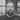# Backpropagation for deep neural networks

6 min read · tagged notes

## Contents

### Feed forward

I’m going to use the notation $\theta_{jk}^{(l)}$ to indicate the weight of the connection from neuron $k^{th}$ in layer $(l - 1)^{th}$ to neuron $j^{th}$ in layer $l^{th}$.

The activation $a_{j}^{(l)}$ for the neuron $j^{th}$ in layer $l^{th}$ is going to be:

$a_j^{(l)} = g(\sum_k \theta_{jk}^{(l)} a_k^{(l-1)})$

Where $a_0^{(l)} = 1$ for every level, to account for the bias term.

For example, you can have:

$\begin{matrix} a_1^{(2)} = g(\theta_{10}^{(2)}x_0 + \theta_{11}^{(2)}x_1 + \theta_{12}^{(2)}x_2 + \theta_{13}^{(2)}x_3) \cr a_2^{(2)} = g(\theta_{20}^{(2)}x_0 + \theta_{21}^{(2)}x_1 + \theta_{22}^{(2)}x_2 + \theta_{23}^{(2)}x_3) \cr a_3^{(2)} = g(\theta_{30}^{(2)}x_0 + \theta_{31}^{(2)}x_1 + \theta_{32}^{(2)}x_2 + \theta_{33}^{(2)}x_3) \end{matrix}$

So you can write in vectorized form as:

$\mathbf{a}^{(l)} = g(\Theta^{(l)} \mathbf{a}^{(l-1)})$

When calculating the activation for one layer, you first need to calculate the quantity:

$\mathbf{z}^{(l)} = \Theta^{(l)} \mathbf{a}^{(l-1)}$

This is called the weighted input to the neurons in layer $l$.

So much for the feed-forward phase.

### Cost function

We can think of neural networks as a class of parametric non-linear functions from a vector $\vec{x}$ of input variables to a vector $\vec{y}$ of output variables. So we can find weights (parameters) as in polynomial curve fitting by minimizing the sum-of-squares error function. So we define a cost function J as

$J(\mathbf{w}) = \frac{1}{2} \sum_{n=0}^N {\parallel \mathbf{y}(\mathbf{x_n},\mathbf{w}) - \mathbf{t_n} \parallel}^2$

Where N is the number of training examples, $\mathbf{t_n}$ is the desired output for the training sample n, and $\mathbf{y}(\mathbf{x_n},\mathbf{w})$ is the calculated output for the corresponding sample. Notice as the cost function is considered dependent only on weights and not on inputs and/or ground truth, as they are given and cannot be changed.

To use the gradient descend, we need to calculate the partial derivatives $\frac{\partial J_n}{\partial w}$ for a single training example and then recover the $\frac{\partial J}{\partial w}$ by averaging over the entire training set. The backpropagation algorithm will be used to calculate such derivatives.

### Backpropagation

#### Errors

Consider the idea to change the weighted input for the neuron $j^{th}$ in layer $l$ of a small quantity $\Delta z_j^{(l)}$. The neuron will output $g(z_j^{(l)} + \Delta z_j^{(l)})$ instead of $g(z_j^{(l)})$, causing an overall change to cost J of the amount $\frac{\partial J}{\partial z_j^{(l)}} \Delta z_j^{(l)}$

If the rate of change of the cost w.r.t. $z_j^{(l)}$ is low, then for a small $\Delta z_j^{(l)}$ the cost won’t change too much. In this case, we say the neuron is nearly optimal. So the quantity $\frac{\partial J}{\partial z_j^{(l)}}$ measures, somehow, how much the neuron is not optimized, and we call it the error $\delta_j^{(l)}$ of the neuron. So by definition, we have:

$\delta_j^{(l)} \equiv \frac{\partial J}{\partial z_j^{(l)}}$

Varying $z_j^{(l)}$ while keeping all other things fixed has some repercussions on the next layer. For a neuron k in layer (l + 1) one can write using the chain rule:

$\frac{\partial J}{\partial z_j^{(l)}} |_k = \frac{\partial J}{\partial z_k^{(l+1)}} \frac{\partial z_k^{(l+1)}}{\partial z_j^{(l)}}$

Which is to say: the contribution of neuron k at the rate of change of cost J caused by neuron j (in the previous layer) is how the cost changes with regards to the weighted sum of neuron k, multiplied for how that said weighted sum changes with regards to the weighted sum of neuron j (chain rule differentiation of function composition).

We can then sum up all the contributions at the level l+1 and state the following:

$\frac{\partial J}{\partial z_j^{(l)}} = \sum_k \frac{\partial J}{\partial z_k^{(l+1)}} \frac{\partial z_k^{(l+1)}}{\partial z_j^{(l)}}$

This is the most important equation of backpropagation, as it put in relation the error $\delta_j^{(l)}$ with the errors in the next layer. We can rewrite it as:

$\frac{\partial J}{\partial z_j^{(l)}} \equiv \delta_j^{(l)} = \sum_k \delta_k^{(l+1)} \frac{\partial z_k^{(l+1)}}{\partial z_j^{(l)}}$

Regarding the quantity $\frac{\partial z_k^{(l+1)}}{\partial z_j^{(l)}}$, we can calculate it starting from the following equation:

$z_k^{(l+1)} = \sum_j \theta_{kj}^{(l+1)} a_j^{(l)} = \sum_j \theta_{kj}^{(l+1)} g(z_j^{(l)})$

If we differentiate w.r.t. $z_j^{(l)}$ we obtain

$\frac{\partial z_k^{(l+1)}}{\partial z_j^{(l)}} = \theta_{kj}^{(l+1)} g\prime(z_j^{(l)})$

So we can finally write

$\delta_j^{(l)} = \sum_k \delta_k^{(l+1)} \theta_{kj}^{(l+1)} g\prime(z_j^{(l)})$Stefano software developer

...